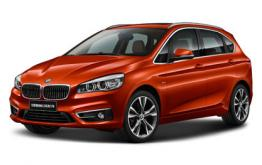# 华晨宝马 宝马2系旅行车

## 日供17元 宝马2系即刻开回家

2016年10月18日 14:13 来源：www.chextx.com 超过：8016次关注

你敢不敢相信，Baby的一辆宝宝推车，就=买一辆BMW车！
辣妈们的一支专属口红，就=买一辆车！
而潮爸的一条品牌领带，也=买一辆车！
超贴心的BMW金融礼遇，就是为时尚的你们量身定制！现在起，购置创新BMW 2系旅行车即有多种金融方案供你选择，有了家庭，依然让你酷劲十足，又可轻松入手，可谓2全其美！

日供低至17元，每天少喝一杯咖啡，BMW 2系即刻开回家！不骗你，现在创新BMW 2系旅行车首付比例40%，贷款分24期，更有每年一次的灵活还款，分摊期末尾款一次性还款压力，并且还款金额可以根据实际情况灵活调整，有效提高你的资金利用率，对于刚刚建立起家庭的你，既可以效优化你的开销，又可以自由自在容纳全家的幸福！以创新BMW 2系旅行车218i为例，首付需支付94,760元，贷款24个月，每年进行一次年度还款，年度还款69,649元，月供低至499元，日供仅需17元！轻松效优化资金运用，每天一杯咖啡钱，即刻把创新BMW 2系旅行车开回家，带着爱人孩子兜风！你更倾向于稳定一些的贷款方式？BMW悦贷金融方案最懂你，除了让你享受低至20%起的首付之外，尾款还款方式的多样化，更可以让你灵活还款，从容购车没压力。24至48个月的自由贷款期，利率低至0.88%，弹性余款更可灵活调节比例，更有新车置换、余款延期、或是一次性还清3种还款方式让你选择，想怎么还就怎么还！

以创新BMW 2系旅行车218i为例，首付只需支付47,380元，贷款分36期，每月月供3,609元，日供仅需120元，尾款还款方式可以根据实际情况选择新车置换、余款延期、或是一次性还清，方式灵活多样，不必担心尾款！想博得一家人的欢心，只有悠贷金融方案怎么能够？现在，根据购车贷款分期的时间，BMW月供增容计划更可以在方方面面给爱车最贴心的保护！同样以创新BMW 2系旅行车218i为例，贷款24期，以支付2年的车辆保险为例，相比之前贷款提车时需要全款支付保险，现在只需在悠贷金融计划的基础上，每日增加1元钱，将车辆保险、甚至细微到钥匙的保障也可一同包含进行分期，即可以超低月供将创新BMW 2系旅行车轻松开回家，更可以给TA全面呵护！(以上保险金额仅供参考)

就这样轻轻松松拥有一台家庭梦想座驾，一年下来，你也许会发现手头资金还绰绰有余，或许还可以去做点投资，添置更好的领带更好的口红，给宝宝更好的座椅。TA是辣妈们的心头之爱，是孩子们的移动城堡，是潮爸们的守护幸福的法宝，快快带上老婆孩子，跟随创新BMW 2系旅行车，这场期待已久的自驾游，说走就走，即刻起程！

#### 相关文章

0-500 字已有评论 0条 查看评论>>

### 宝马2系旅行车### 热门标签

﻿
• 快速找车
• 选择品牌
• 选择品牌
• A  奥迪
• A  阿斯顿·马丁
• A  阿尔法·罗密欧
• B  宝沃
• B  布加迪
• B  巴博斯
• B  保时捷
• B  宾利
• B  奔驰
• B  宝马
• B  本田
• B  别克
• B  标致
• B  比亚迪
• B  宝骏
• B  北汽制造
• B  北汽新能源
• B  北汽幻速
• B  北汽威旺
• B  北京汽车
• B  奔腾
• B  北汽绅宝
• C  长安
• C  长安商用
• C  长城
• C  昌河
• D  大众
• D  道奇
• D  DS
• D  东南
• D  东风风神
• D  东风风行
• D  东风小康
• D  东风风度
• D  东风
• F  福特
• F  丰田
• F  菲亚特
• F  法拉利
• F  福田
• F  福迪
• F  福汽启腾
• G  观致
• G  广汽传祺
• G  广汽吉奥
• G  GMC
• H  红旗
• H  汉腾汽车
• H  哈弗
• H  哈飞
• H  海格
• H  海马
• H  华颂
• H  黄海
• H  华泰
• H  恒天
• J  吉利汽车
• J  捷豹
• J  Jeep
• J  江淮
• J  江铃
• J  金杯
• J  九龙
• J  金旅
• K  凯翼
• K  凯迪拉克
• K  克莱斯勒
• K  科尼塞克
• K  卡威
• K  开瑞
• L  路虎
• L  林肯
• L  劳斯莱斯
• L  兰博基尼
• L  雷克萨斯
• L  铃木
• L  雷诺
• L  理念
• L  力帆
• L  莲花汽车
• L  猎豹
• L  路特斯
• L  陆风
• M  马自达
• M  MG
• M  MINI
• M  玛莎拉蒂
• M  摩根
• M  迈凯轮
• N  纳智捷
• O  欧宝
• O  讴歌
• O  欧朗
• Q  奇瑞
• Q  起亚
• Q  启辰
• R  日产
• R  荣威
• R  瑞麒
• S  三菱
• S  斯威汽车
• S  萨博
• S  smart
• S  斯柯达
• S  斯巴鲁
• S  思铭
• S  双龙
• S  上汽大通
• S  双环
• T  特斯拉
• T  腾势
• W  沃尔沃
• W  五菱汽车
• W  五十铃
• W  威兹曼
• W  威麟
• X  现代
• X  雪佛兰
• X  雪铁龙
• X  西雅特
• Y  一汽
• Y  英菲尼迪
• Y  英致
• Y  依维柯
• Y  野马汽车
• Y  永源
• Z  众泰
• Z  中华
• Z  中兴
• Z  知豆
• 选择车系
• 选择车系
• 车型对比
• 选择品牌
• 选择品牌
• A  奥迪
• A  阿斯顿·马丁
• A  阿尔法·罗密欧
• B  宝沃
• B  布加迪
• B  巴博斯
• B  保时捷
• B  宾利
• B  奔驰
• B  宝马
• B  本田
• B  别克
• B  标致
• B  比亚迪
• B  宝骏
• B  北汽制造
• B  北汽新能源
• B  北汽幻速
• B  北汽威旺
• B  北京汽车
• B  奔腾
• B  北汽绅宝
• C  长安
• C  长安商用
• C  长城
• C  昌河
• D  大众
• D  道奇
• D  DS
• D  东南
• D  东风风神
• D  东风风行
• D  东风小康
• D  东风风度
• D  东风
• F  福特
• F  丰田
• F  菲亚特
• F  法拉利
• F  福田
• F  福迪
• F  福汽启腾
• G  观致
• G  广汽传祺
• G  广汽吉奥
• G  GMC
• H  红旗
• H  汉腾汽车
• H  哈弗
• H  哈飞
• H  海格
• H  海马
• H  华颂
• H  黄海
• H  华泰
• H  恒天
• J  吉利汽车
• J  捷豹
• J  Jeep
• J  江淮
• J  江铃
• J  金杯
• J  九龙
• J  金旅
• K  凯翼
• K  凯迪拉克
• K  克莱斯勒
• K  科尼塞克
• K  卡威
• K  开瑞
• L  路虎
• L  林肯
• L  劳斯莱斯
• L  兰博基尼
• L  雷克萨斯
• L  铃木
• L  雷诺
• L  理念
• L  力帆
• L  莲花汽车
• L  猎豹
• L  路特斯
• L  陆风
• M  马自达
• M  MG
• M  MINI
• M  玛莎拉蒂
• M  摩根
• M  迈凯轮
• N  纳智捷
• O  欧宝
• O  讴歌
• O  欧朗
• Q  奇瑞
• Q  起亚
• Q  启辰
• R  日产
• R  荣威
• R  瑞麒
• S  三菱
• S  斯威汽车
• S  萨博
• S  smart
• S  斯柯达
• S  斯巴鲁
• S  思铭
• S  双龙
• S  上汽大通
• S  双环
• T  特斯拉
• T  腾势
• W  沃尔沃
• W  五菱汽车
• W  五十铃
• W  威兹曼
• W  威麟
• X  现代
• X  雪佛兰
• X  雪铁龙
• X  西雅特
• Y  一汽
• Y  英菲尼迪
• Y  英致
• Y  依维柯
• Y  野马汽车
• Y  永源
• Z  众泰
• Z  中华
• Z  中兴
• Z  知豆
• 选择车系
• 选择车系
• 选择车型
• 选择车型
• 意见反馈